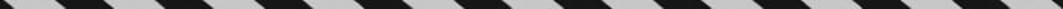﻿ ﻿Exercises - Fractions, Decimals, and Percents - TOPICS IN ARITHMETIC - SAT SUBJECT TEST MATH LEVEL 1

## TOPICS IN ARITHMETIC## CHAPTER 3Fractions, Decimals, and Percents### Exercises1. Which of the following is not an irrational number?

(A)(B)(C)(D)(E)2. What fraction of 72 isof 80?

(A)(B)(C)(D)(E)3. Which of the following lists the numbersandin increasing order?

(A)(B)(C)(D)(E)4. If 0 < x < 1, which of the following could be less than x?

I. x2

II. 10x % of x

III.(A) I only

(B) II only

(C) III only

(D) I and II only

(E) I and III only

5. Which of the following numbers satisfies the inequality?

(A)(B)(C)(D)(E)6. If 5x = 3 and 3y = 5, what is the value of?

(A)(B)(C) 1

(D)(E)7. What percent of 50 is w?

(A) 2w

(B)(C)(D)(E)8. At Sally’s Sale Shop everything is sold for 20% less than the price marked. If Sally buys sweaters for \$80, what price should she mark them if she wants to make a 20% profit on her cost?

(A) \$80

(B) \$96

(C) \$100

(D) \$120

(E) \$125

9. From 1990 to 2000, the number of students attending McKinley High School increased by 3.5% each year. If the school had 800 students in 1990, how many did it have in 2000?

(A) 1,080

(B) 1,090

(C) 1,128

(D) 1,168

(E) 1,215

10. On a test consisting of 80 questions, Marie answered 75% of the first 60 questions correctly. What percent of the other 20 questions did she need to answer correctly for her grade on the entire exam to be 80%?

(A) 85%

(B) 87.5%

(C) 90%

(D) 95%

(E) 100%

 1. (D) 5. (D) 8. (D) 2. (E) 6. (A) 9. (C) 3. (B) 7. (A) 10.(D) 4. (D)

Solutions

Each of the problems in this set of exercises is typical of a question you could see on a Math 1 test. When you take the model tests in this book and, in particular, when you take the actual Math 1 test, if you get stuck on questions such as these, you do not have to leave them out—you can almost always answer them by using one or more of the strategies discussed in the “Tactics” chapter. The solutions given here do not depend on those strategies; they are the correct mathematical ones.

See Important Tactics for an explanation of the symbol ⇒, which is used in several answer explanations.

1. (D), which is a rational number. It is not irrational. Choices A, B, and C are each equal to, which is irrational. Choice E is also irrational since, and the reciprocal of an irrational number is irrational.

2. (E)of 80 =80 = 48, and 48 isof 72.Finally,.

3. (B) Use your calculator to convert each fraction to a decimal.This is the correct order.

4. (D)(I is true).(II is true).(III is false).

Only I and II are true.

5. (D) Convert the fraction to decimals. Then the inequality becomes. There are infinitely many values ofthat satisfy this inequality, butis one obvious choice. Then.

6. (A)and. So.

7. (A).

8. (D) Since 20% of 80 is 16, Sally wants to get \$80 + \$16 = \$96 for each sweater she sells. Then if x is the marked price,9. (C) The number of students in the school in 2000 was (1.035)10(800) = 1,128.10. (D) To earn an 80% on the entire exam, Marie needs to answer a total of 64 questions correctly (80% of 80). So far, she has answered 45 questions correctly (75% of 60). Therefore, on the last 20 questions, she needs 64 – 45 =19 correct answers, and.

﻿